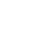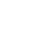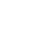# Resistors in Series and Parallel

The average person uses a lot of electricity in the many things they do every day. We are used to being able to turn on a light, watch the television, use our mobile devices, and stream music or videos. Because electricity is so much a part of our lives, we should know something about it.

### Current flow and Nodes

To understand series circuits you need to know a little about nodes. A node is just the electrical junction that exists between two (or more) components. When someone draws circuitry schematics, they show where the nodes are by displaying where the wires are between the components.Another important thing to know is to be able to understand that current flows through a circuit from high voltage to lower voltage. Some of the current goes through every path that it can find to finally get to the lowest voltage point (normally called the “ground.”)

### Series Circuits

A series circuit allows the current to follow one path. It’s also called a “closed circuit.” In a series circuit the current through each load is the same. A series circuit also has the total voltage across the circuit as the same as the sum of the voltages across each of the loads.

When creating electronic circuits we can use resistors in a combination of ways. To figure out the resistance of a circuit or even some of the circuit, you need to see which resistors are in a series (one path) or in parallel (more than one path.)

### Series resistors

When you connect resistors end-to-end in a circuit, they are said to be in a series. To find the total resistor resistance in a series you only need to add up the value of each of the resistors.

### Parallel circuits

A parallel circuit is where the circuit is divided into two or more paths for the flow of electricity.

### Parallel resistors

Parallel resistors are the resistors that are connected across from each other in an electric circuit.

To get the series resistance we added up all of the resistance from the resistors. However, for parallel resistors we know that some of the current travels through one resistor, more of it through another resistor and even some to a possible third.

Each resistor gives another path for the current to flow. In an electronic circuit with three parallel resistors we will have to use the formula before to figure out the total resistance that is happening across the voltage:

In the case of parallel resistors, the total resistance is less than any of the resistors that are in parallel and always be less than the smallest resistor that is in parallel.

### Equivalent Resistance

The more resistance that you have in electronic circuitry the less of a flow of current through the circuit.

Equivalent resistance is just another way of saying “total resistance.” This is commonly referred to and used when we have to calculate combined series and parallel circuits.

### Series and Parallel, Together

There is circuitry that has a combination of both series and parallel resistors. This happens in more sophisticated electronic circuitry. The way to figure out these kinds of circuits is to first break them down to their individual series and parallel circuits or their smaller parts.

Start with the series resistors and replace them with the “equivalent resistance.” The next step is to do the parallel areas and replace those with equivalent resistors. Keep doing the math until you reach the end and you will have your answer.

### Q&A

What is a node?
the electrical junction that exists between two (or more) components

Current flows through a circuit in what voltage manner?
high to low

What is the lowest voltage point in a circuit usually called?
ground

What is a series circuit?
A series circuit allows the current to follow one path

What is a parallel circuit?
A parallel circuit is where the circuit is divided into two or more paths for electricity flow

Where would you find parallel resistors in electrical circuitry?
they are opposite or across from each other

What is another term for equivalent resistance?
total resistance

•History
•Science
•Geography
•Biography
•US History